Catch rate

(Redirected from Catch)This article is incomplete.Please feel free to edit this article to add missing information and complete it. Reason: Capture method and modifiers for Generation VII and all information for Generation VIII

Catch redirects here. For the concept of catching a Pokémon, see Caught Pokémon.

Each species of Pokémon has a catch rate that applies to all its members. When a Poké Ball is thrown at a wild Pokémon, the game uses that Pokémon's catch rate in a formula to determine the chances of catching that Pokémon. Higher catch rates mean that the Pokémon is easier to catch, up to a maximum of 255. The formula also takes into account the following factors:

Other factors, such as the Pokémon's level and encounter rate, have no bearing on its chances of being caught.

Poké Balls that guarantee capture (the Master Ball and Park Ball, and any Poké Ball used on Kalos Route 2XY, Route 101ORAS, Alola Route 1SMUSUM prior to the start of the festival in Iki Town, or in the Entree Forest) bypass the catching formula entirely.

Capture method (Generation I)

The capture method in Generation I differs significantly from those of later generations. To determine whether a Pokémon is caught or not, the steps below are performed. If, at any point, the Pokémon is caught or breaks free, the steps following that point are not performed.

1. If a Master Ball is used, the Pokémon is caught.
2. Generate a random number, N, depending on the type of ball used.
3. The Pokémon is caught if...
• It is asleep or frozen and N is less than 25.
• It is paralyzed, burned, or poisoned and N is less than 12.
4. Otherwise, if N minus the status threshold (above) is greater than the Pokémon's catch rate, the Pokémon breaks free.
5. If not, generate a random value, M, between 0 and 255.
6. Calculate f:
• $f=\left\lfloor {\dfrac {HP_{max}\times 255\times 4}{HP_{current}\times Ball}}\right\rfloor$, where the value of Ball is 8 if a Great Ball is used or 12 otherwise. The minimum value of f is 1 and its maximum value is 255.
7. If f is greater than or equal to M, the Pokémon is caught. Otherwise, the Pokémon breaks free. In practical terms, lowering the target's HP to 1/3 of its maximum will guarantee capture with a Poké Ball, while lowering it to 1/2 will guarantee capture with a Great Ball.

If the Pokémon broke free, the steps below are performed to determine how many times the ball will shake.

1. Calculate d:
• $d=\left\lfloor {\dfrac {catchRate\times 100}{Ball}}\right\rfloor$, where the value of Ball is 255 for the Poké Ball, 200 for the Great Ball, or 150 for other balls.
2. If d is greater than or equal to 256, the ball shakes three times before the Pokémon breaks free.
3. If not, calculate $x=\left\lfloor {\dfrac {d\times f}{255}}\right\rfloor +s$, where s is 10 if the Pokémon is asleep or frozen or 5 if it is paralyzed, poisoned, or burned.
4. If...
• x < 10: the Ball misses the Pokémon completely.
• x < 30: the Ball shakes once before the Pokémon breaks free.
• x < 70: the Ball shakes twice before the Pokémon breaks free.
• Otherwise, the Ball shakes three times before the Pokémon breaks free.

This algorithm thus has several curious properties that do not apply in later generations:

• The amount of times a Poké Ball shakes before it breaks free (or not at all if it misses the Pokémon) is a rough approximation of the Pokémon's catch odds.
• If a Pokémon will break free, a given combination of ball plus status condition plus HP will always result in the same number of shakes. This means that, if a given number of shakes is observed before the Pokémon breaks free for a given combination of these values, if the ball is ever observed to shake more times with the same combination of values, the Pokémon will be caught.
• Under certain situations, a Great Ball is more effective than an Ultra Ball. In particular, Pokémon with high catch rates, no status, and above ½ HP may guarantee capture with a Great Ball but fail to do so with an Ultra Ball.
• The effect of reducing HP diminishes with lower catch rates, and the effect of inflicting status diminishes with higher catch rates. Regardless of catch rate, reducing the HP does not increase chances of capture at less than ½ HP with Great Balls, and ⅓ HP with other balls.
• Inflicting a status ailment gives the player a flat additional chance (from 4.6% to 16.6%) to catch the Pokémon regardless of all other factors, making certain Pokémon easier to catch in Generation I than they are in later generations: A sleeping Mewtwo with full HP may, on average, be caught in about six Ultra Balls in Generation I, but from Generation II onwards exactly the same circumstances would require an average of about 64 Ultra Balls— over ten times as many.
• Due to the nature of the algorithm, Ultra Balls will only perform better than Great Balls on Pokémon whose capture rates are above 55 and below 200 in Generation I. Ultra Balls increase the overall chance of capture by as much as 20% in comparison to Great Balls for Pokémon near the center of that range.
• Assuming the current HP of target Pokémon is less than or equal to ½ HP for Great Balls and ⅓ HP for Ultra Balls, Ultra Balls increase the overall chance to capture by 10% or more in comparison to Great Balls for the following Pokémon:
- Metapod, Kakuna, Pidgeotto, Raticate, Fearow, Arbok, Sandslash, Nidorina, Nidorino, Clefairy, Jigglypuff, Golbat, Gloom, Persian, Poliwhirl, Kadabra, Machoke, Weepinbell, Graveler, Haunter, Exeggcute, and Rhyhorn.

Approximate probability

The following calculation will give the exact probability of catching a Pokémon whose catch rate is at most a specific value. It will give results which are too high if the catch rates are more than this, but it remains a useful approximation. Specifically:

• For a Poké Ball, it is exact for Pokémon with a catch rate of at most 230;
• For a Great Ball, it is exact for Pokémon with a catch rate of at most 175
• For an Ultra or Safari Ball, it is exact for Pokémon with a catch rate of at most 125.

$CaptureProbability\approx p_{0}+p_{1}$where

$p_{0}={\dfrac {statusAilment}{ballMod+1}}$• statusAilment = 12 if poisoned, burned, or paralyzed, 25 if frozen or asleep, 0 otherwise.
• ballMod = 255 if using a Poké Ball, 200 if using a Great Ball, and 150 otherwise.

and

$p_{1}={\dfrac {catchRate+1}{ballMod+1}}\times {\dfrac {f+1}{256}}$• f is defined in the above section for the capture method.
• catchRate (given as an integer value) is stated on each individual Pokémon's article.

General capture method (Generation II onwards)

The capture algorithms in Generation II and onwards have three essential components: the modified catch rate, the "shake probability", and the "shake checks". Generation II handles shake checks slightly differently than the later generations.

Modified catch rate

The modified catch rate, a, is the catch rate after various factors such as weakening the Pokémon and using stronger Poké Balls are taken into consideration. A modified catch rate may never fall to 0 (that is, render a Pokémon impossible to capture), but it may cause the modified rate to fall below its original unmodified catch rate (such as from high health, Heavy Balls, Baiting in the Safari Zone, or the dark grass penalty in Generation V). In Generation III and Generation IV, the modified catch rate may never fall below 1.

Shake probability

The shake probability, b, is a value that determines the probability that a single shake check passes.

Shake checks

Shake checks are performed to determine whether the Pokémon will be caught or, if the Pokémon breaks free, the number of shakes that will occur before it does so.

In Generation II, whether a Pokémon will be caught is determined before any shake checks are performed, and shake checks are only performed if the Pokémon is not caught.

Capture method (Generation II)

Modified catch rate

The modified catch rate a is calculated in Generation II as follows:

$a=\mathrm {max} \left(\left\lfloor {\dfrac {(3\times HP_{max}-2\times HP_{current})\times rate_{modified}}{3\times HP_{max}}}\right\rfloor ,1\right)+bonus_{status}$where

• HPmax is the number of hit points the Pokémon has at full health,
• HPcurrent is the number of hit points the Pokémon has at the moment,
• ratemodified is the catch rate of the Pokémon modified by the ball used, but no less than 1 and no more than 255,
• bonusstatus is the modifier for any status condition the Pokémon has (10 for sleep or freeze, 0 otherwise).
• bonusstatus was intended to equal 5 for paralyze, poison, or burn, but due to a glitch, the game skips this check.

If 3 × HPmax > 255, then both 3 × HPmax and 2 × HPcurrent are halved twice (and rounded down after each division) for use in the formula, as the values used are unsigned 8-bit integers. If the latter product is 0, it is set to 1 instead.

If the Pokémon's HP is 342 or greater, the 3 × HPmax value will be truncated and the subtraction may underflow, giving bizarre results and even making it possible for the game to freeze; however, no such Pokémon can be legitimately encountered in-game.

a is capped at 255.

Shake probability

The shake probability b is determined from the table below, depending on the value of a.

 a b 0-1 2 3 4 5 6-7 8-10 11-15 16-20 21-30 31-40 41-50 51-60 61-80 81-100 101-120 121-140 141-160 161-180 181-200 201-220 221-240 241-254 255 63 75 84 90 95 103 113 126 134 149 160 169 177 191 201 211 220 227 234 240 246 251 253 255

Shake checks

First, a check is performed to determine whether the Pokémon is caught at all. A random number between 0 and 255 is generated, and if this number is less than or equal to a, the Pokémon is caught.

Shake checks are only performed if the Pokémon is not caught. A single shake check consists of generating a random number between 0 and 255 and comparing it to b. This is done at most three times, but if the number generated in a given shake check is greater than or equal to b, no further shake checks will be performed. The number of times the ball shakes is the same as the number of shake checks that were performed. The table is essentially a very low-precision lookup table with numbers corresponding to the shake rate in later generations, so ultimately, aside from rounding errors (very bad ones in this case), the shake and capture rate remain similar between Generation II and the formula later used in Generation III.

Capture method (Generation III-IV)

Modified catch rate

The modified catch rate, a, is calculated in Generation III and Generation IV as follows:

$a={\dfrac {(3\times HP_{max}-2\times HP_{current})\times rate\times bonus_{ball}}{3\times HP_{max}}}\times bonus_{status}$Where

• HPmax is the number of hit points the Pokémon has at full health,
• HPcurrent is the number of hit points the Pokémon has at the moment,
• rate is the catch rate of the Pokémon (which may be modified due to use of apricorn balls or actions in the Safari Zone),
• bonusball is the multiplier for the Poké Ball used, and
• bonusstatus is the multiplier for any status condition the Pokémon has (2 for sleep and freeze, 1.5 for paralyze, poison, or burn, and 1 otherwise). Due to a bug in Ruby & Sapphire, if the target Pokémon is afflicted with Toxic Poison, no catch bonus is applied. This was fixed in FireRed and LeafGreen.

If a Pokémon could have 0 HP, the maximum value for a would be rate × bonusball × bonusstatus. For a Pokémon with full health and no status condition, and with a neutral ball used, the minimum value for a would be rate / 3.

The formula is slightly different when applied to the Apricorn balls in HeartGold and SoulSilver. The modifiers for these balls are applied directly to the Pokémon's catch rate, rather than in the formula. In this case, bonusball is always 1, and the catch rate cannot go higher than 255. This means that for Pokémon whose catch rate is already 255, such as Rattata, the Apricorn balls do not make the capture any more likely than a regular Poké Ball.

Shake probability

The shake probability is calculated as follows:

$b={\dfrac {1048560}{\sqrt {\sqrt {\dfrac {16711680}{a}}}}}$The divisions and square roots all round down to the nearest integer.

Shake checks

To perform a shake check, a random number between 0 and 65535 (inclusive) is generated and compared to b. If the number is greater than or equal to b, the check "fails".

Four shake checks are performed. The Pokémon is caught if all four shake checks succeed. Otherwise, the Poké Ball will shake as many times as there were successful shake checks before the Pokémon breaks free.

In Pokémon Colosseum and Pokémon XD: Gale of Darkness, the Pokémon breaks free after shaking once if the first or second check fails. Otherwise, the Poké Ball will shake as many times as there were successful shake checks before the Pokémon breaks free. A different message will appear depending on if the first or second check fails, despite the Poké Ball always shaking at least once.

If a is 255 or greater, the capture will always succeed and no shake checks will be performed.

Probability of capture

The probability p of catching a Pokémon—given the values a and b calculated above for each shake, then requiring all 4 shakes be passed—is approximately ${\textstyle \left({b \over 65535}\right)^{4}}$, or simply ${\textstyle a \over 255}$. Due to rounding errors produced when calculating b, this approximation can be significantly inaccurate; for example, all a values greater than 200 yield the same b value, 65535, which results in a 99.994% chance of a successful capture.

For a constant capture probability p, the probability ${\textstyle P(r)}$that a player can capture the Pokémon with no more than r tries is:

$P(r)=1-(1-p)^{r}$Note that this is the cumulative probability function for the random variable r, which has a geometric distribution. The expected value of the random variable r is 1/p, that is to say, on average, a Pokémon that can be caught with probability p will be caught with 1/p tries.

The inverse problem, the number of tries, r, needed to have a probability P of capturing a Pokémon is:

$r={\dfrac {log(1-P)}{log(1-p)}}$Capture method (Generation V)

Modified catch rate

Generation V follows the formula in Generation III-IV, with all divisions above rounded down to the nearest multiple of 1/4096. However, there are three alterations:

• bonusstatus is now 2.5 for sleep and freeze (instead of 2).
• Capture Power factor: if a Capture Power is active, the catch rate is multiplied by a factor and rounded down to the nearest multiple of 1/4096.
• Capture Power ↑: ×1.1
• Capture Power ↑↑: ×1.2
• Capture Power ↑↑↑, S, or MAX: ×1.3
• Dark grass factor: This factor is multiplied into the HP factor (${\textstyle 3\times HP_{max}-2\times HP_{current}}$above) and then rounded to the nearest multiple of 1/4096. This factor is applied if the battle occurs in dark grass, and depends on the number of Pokémon that have been caught in the Pokédex, as shown in the table below, usually making it harder to catch a Pokémon. This even occurs when only one Pokémon is met in the dark grass.
Number Caught Multiplier
> 600 1
451-600 3686/4096 (90%)
301-450 3277/4096 (80%)
151-300 2867/4096 (70%)
30-150 2048/4096 (50%)
< 30 1229/4096 (30%)

If the modified catch rate is greater than 255, the Pokémon is guaranteed to be caught (but a critical capture check will be performed anyway).

Shake probability

The formula for b is

$b={\Biggl \lfloor }{\dfrac {65536}{\sqrt {\sqrt {\dfrac {255}{a}}}}}{\Biggr \rfloor }$where the divisions and square roots are rounded to the nearest 1/4096

Shake checks

Generation V's shake checks work essentially the same as those of Generations III and IV.

Before any shake checks are performed, the game checks to see if a critical capture may happen. If not, three shake checks will be performed, otherwise, only one shake check will be performed.

In a normal capture, the Pokémon breaks free without shaking if the first check fails or after one shake if the second check fails. If the third check fails, the Pokémon breaks free after three shakes (not two).

In a critical capture, the ball will always shake once, and the Pokémon will break free or be caught depending on the result of the shake check.

Capture method (Generation VI)

In Generation VI games, on the first route where a player can catch wild Pokémon (Kalos Route 2 in Pokémon X and Y or Hoenn Route 101 in Pokémon Omega Ruby and Alpha Sapphire), wild Pokémon will never break out of a Poké Ball thrown at them.

Modified catch rate

The modified catch rate is essentially identical in all respects to Generation V, except that Capture O-Powers give a 1.5×, 2× or 2.5× boost rather than 1.1×, 1.2× and 1.3×.

Shake probability

Unlike Generation V, the shake probability in Generation VI is calculated as follows:

$b={\dfrac {65536}{\left({\dfrac {255}{a}}\right)^{0.1875}}}$Shake checks

The Generation VI games perform four shake checks again, like Generations III-IV. Critical captures function like in Generation V, with only one shake check being performed for a critical capture.

The change to the formula for the shake probability is designed to counteract the change in the number of shake checks: the odds of success for a normal capture are the same in Generation VI as in Generation V, barring small rounding errors. Since each shake check is more likely to succeed, however, critical captures are more effective than before.

Critical captureThis section is incomplete.Please feel free to edit this section to add missing information and complete it. Reason: Pokémon GO and Catching Charm chances

Since Generation V, when a Poké Ball is thrown, a special type of capture that is much more likely to catch a Pokémon, called a critical capture (Japanese: 捕獲クリティカル critical capture), may occur. When a critical capture occurs, the Poké Ball will make a high-pitched whistling sound as it is thrown, then pause in mid-air, and shudder before it drops down to the ground, shaking only once on the ground before the Pokémon escapes or is caught.

The probability of a critical capture occurring depends of the number of Pokémon that have been caught in the Pokédex. First, compute the value of a (the modified catch rate) as shown in the appropriate section above. Then, multiply a by the appropriate multiplier from the table below.

Species Caught Multiplier
>600 2.5
451-600 2
301-450 1.5
151-300 1
31-150 0.5
≤30 0

Finally, divide the result by 6 and round down to the nearest integer. Call this final result c. A random number between 0 and 255 is generated; if it is less than c, a critical capture will occur. Overall, this means the critical capture chance ranges from 0 (if 30 or fewer species have been caught) to about 41.67% of the modified capture rate a (if over 600 species have been caught).

Critical captures only make one shake check instead of three or four, which means they are considerably more likely to successfully capture the Pokémon. In Generation V, the success chance for a critical capture is the cube root of the success chance for a regular capture; thus, for example, if the regular success chance is 5%, the chance of success with a critical capture will be about 37%, while if the regular success chance is 50%, the chance of success with a critical capture will be just over 79%. In Generation VI, meanwhile, the success chance for a critical capture is instead the fourth root of the success chance for a regular capture: if the regular success chance is 5%, the critical success chance will be around 47%, while if the regular success chance is 50%, the critical success chance will be around 84%. Given the rarity of critical captures, however, the Pokémon is always more likely overall to be caught with a regular capture than a critical one.

Poké Ball effectiveness rates

Ball Rate NotesPoké BallGreat Ball 1.5×Ultra BallMaster Ball N/A Never fails, except against unidentified ghosts, the ghost Marowak, Kyurem when under Ghetsis's control, and Nihilego in Aether ParadiseSafari Ball 1.5×Gen I-VII, 1×Gen VIIILevel Ball 1×, 2×, 4× or 8× 1× if the player's Pokémon is the same level as or a lower level than the wild Pokémon

2× if the player's Pokémon is at a higher level than the wild Pokémon but less than double it
4× if the player's Pokémon is more than double but less than four times the level of the wild Pokémon
8× if the player's Pokémon is of a level four times or more than that of the wild PokémonLure Ball 1× or 3×GSCHGSS/5×Gen VII/4×Gen VIII 3×/5×/4× only if fishingMoon Ball 1× or 4× Always 1× due to a glitchGSC
4× only if used on a Pokémon in the Nidoran♂, Nidoran♀, Clefairy, Jigglypuff, Skitty, or Munna families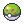Friend BallLove Ball 1× or 8× 8× only if used on a Pokémon of the same species and gender as the player's PokémonGSC

8× only if used on a Pokémon of the same species as, but opposite gender of, the player's PokémonHGSSSMHeavy Ball -20, 0, +20, +30 or +40 -20 if used on Pokémon weighing less than 225.8 lbs.GSC*/451.5 lbs.HGSS*/220.46 lbs.SM*USUM*
No modifier if used on Pokémon weighing between 225.8 lbs.GSC/220.46 lbs.SM and 451.5 lbs.GSC/440.92 lbs.SM
+20 if used on Pokémon weighing between 451.5 lbs.GSCHGSS/440.92 lbs.SM and 677.3 lbs.GSCHGSS/661.38 lbs.SM
+30 if used on Pokémon weighing more than 661.38 lbs.SM
+30 if used on Pokémon weighing between 677.3 lbs. and 903.0 lbs.GSCHGSS
+40 if used on Pokémon weighing more than 903.0 lbs.GSCHGSSFast Ball 1× or 4× 4× only if used on Magnemite, Grimer, or TangelaGSC

4× only if used on a Pokémon with a base Speed of at least 100HGSSSMSport Ball 1.5×Gen II-VII, 1×Gen VIIINet Ball 1× or 3×Gen III-VI/3.5×Gen VII 3×/3.5× only if the target is a Bug- or Water-type PokémonNest Ball 1× to 4× ((40 - Pokémon's level) ÷ 10)×, minimum 1× (reached at level 30)Gen III-IV
((41 - Pokémon's level) ÷ 10)×, minimum 1×Gen V
((41 - Pokémon's level) ÷ 10)× if Pokémon's level is between 1 and 29, 1x otherwiseGen VI+Repeat Ball 1×, 3×Gen III-VI or 3.5×Gen VII 3×/3.5× only if target has been caught previouslyTimer Ball 1× to 4× ((number of turns passed in battle + 10) / 10)×, maximum Gen III-IV
(1 + number of turns passed in battle * 1229/4096)×, maximum Gen V+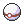Premier BallLuxury Ball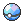Dive Ball 1× or 3.5× 3.5× only if divingRSEORAS or surfing/fishingDPPtHGSSBWB2W2XYSM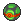Dusk Ball 1× or 3.5×Gen III-VI/3×Gen VII 3.5×/3× only if inside a cave or at night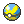Quick Ball 1× or 4×Gen IV/5×Gen V-VII 4×/5× only on the first turn of the battle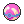Heal BallCherish BallPark Ball N/A Never fails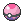Dream Ball N/A (when used in Entree Forest)/1× or 4×Gen VIII Never fails in Generation V; only 4× when used against a sleeping Pokémon in Generation VIII.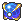Beast Ball 5× or 0.1x 5× only if used on an Ultra Beast
0.1× on everything else

Examples

From Generation III onward, if a Pokémon's HP is almost depleted, the chance of a single ball catching it can be approximated as

$CatchChance\approx {rate\times bonus_{ball}\times bonus_{status} \over 255}$Consider a Pokémon with a catch rate of 3 (which is the case for most Legendary Pokémon) whose almost depleted its HP and is paralyzed.

If a Dusk Ball is used at night or in a cave (Ball bonus of 3.5), then the probability of capture per Ball is approximately ${\textstyle {3\times 3.5\times 1.5 \over 255}={15.75 \over 255}\approx 6.2\%}$. This means that the average number of Dusk Balls required for capture is ${\textstyle {1 \over 6.2\%}\approx 16}$.

If an Ultra Ball were used instead (Ball bonus of 2), then the probability of capture per Ball is approximately ${\textstyle {3\times 2\times 1.5 \over 255}={9 \over 255}\approx 3.5\%}$. This means that the average number of Ultra Balls required for capture is instead ${\textstyle {1 \over 3.5\%}\approx 28}$.

Alternatively, if a Timer Ball is used after the 30th turn (Generation III to IV) or 10th turn (Generation V), resulting in a Ball bonus of 4, the probability of capture per Ball is approximately ${\textstyle {3\times 4\times 1.5 \over 255}={18 \over 255}\approx 7.1\%}$. This means that the average number of Timer Balls required for capture is ${\textstyle {1 \over 7.1\%}\approx 14}$.

Quotes

Shakes Generation I Generation II Generation III Generation IV Generation V Generation VI Generation VII Generation VIII
SMUSUM PE
0 You missed the Pokémon! Oh no! The Pokémon broke free! Oh, no! The Pokémon broke free! Oh, no! The Pokémon broke free! Oh no! The Pokémon broke free! Ah, no! The Pokémon broke free from the Poké Ball! Oh no! The Pokémon broke free!
1 Darn! The Pokémon broke free! Aww! It appeared to be caught! Ahhh! Just when it seemed like you had it! Aww! It appeared to be caught!
2 Aww! It appeared to be caught! Aargh! Almost had it! * Aargh! Almost had it! What a shame! You were so close to catching it, too! Aargh! Almost had it!
3 Shoot! It was so close too! Shoot! It was so close, too! Aargh! Almost had it! Gah! It was so close, too! So close! You almost got it! Gah! It was so close, too!
Caught All right! <Pokémon> was caught! Gotcha! <Pokémon> was caught! All right! You caught a/an <Pokémon>! Gotcha! <Pokémon> was caught!

Other uses

Generation I

Main article: List of Pokémon by wild held item (Generation II)

In the Generation I games, when a Pokémon is caught, its catch rate is saved in its data structure, and remains unchanged even upon evolution. If a Pokémon caught in a Generation I game is traded to a Generation II game, this value becomes the Pokémon's held item.

In Pokémon Yellow and Pokémon Stadium, this value is specifically set for particular Pokémon to ensure that the Pokémon will hold a specific item when traded to a Generation II game.

Trivia

• If used outside of the Entree Forest prior to Generation VIII (which was not possible in regular gameplay), the Dream Ball has a catch rate of 1×.
• The line if a Pokémon escapes at three shakes in Generation III and IV, "Shoot! It was so close, too!", remains in the internal data of the Generation V games despite not being used.
• In Pokémon Sun and Moon, a Heavy Ball will always fail to catch a Pokémon whose weight is less than 220.46 lbs (100.0 kg) and whose catch rate is less than or equal to 20. This is because the game will set the catch rate to 0 when the catch rate becomes negative due to the -20 modifier that is applied. The only catchable Pokémon that this affects are Beldum and the guardian deities. This error was corrected in Pokémon Ultra Sun and Ultra Moon.
• The numbers 255, 4096, and 65536 result from the data types used. 255 is the highest number a byte can encode. 4096 is the amount of space a 12-bit integer can encode. 65536 is the amount of space two bytes can encode.